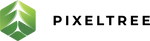Back to Pixeltree
Tech
The difference between let and let! in RSPEC
Carolina Contreras Issa
1 min

When writing RSPEC tests you will most likely run into these two helper methods: let and let! — they seem similar, but are they really?

• When we use ‘let’ we are able to use a memoized helper method
• Memoization == cache. When you use ‘let’ the value that you store in will be cached. This means that — the ‘example’ method will only be created once AND it will only be created when it needs to (lazy loading)
• Lazy loading means that the block will only run if and when it is referenced

Let’s look at the following example:

describe ‘Example 1’ do

let(:example) create(:example)

it ‘increases example by 1’ do

end

it ‘has a title’ do

end

end

describe ‘Example 2 do

it ‘has a subtitle’ do

end

end

• In the example above, the example method that we called will be able to be used in the two ‘it’ statements inside the first describe block but not inside the second describe block - you will need to create a second example method for that one
• Even though you are actually calling a helper method, I like to see ‘let’ as a way to create a variable — it helps me visualize it better
• When we use let! you are calling an implicit before block before the example block like so:

describe ‘Example 1’ do

let(:example) create(:example)

before(:each) do puts ‘this runs before the example’

end

it ‘increases example by 1’ do

end

it ‘has a title’ do

end

end

describe ‘Example 2 do

it ‘has a subtitle’ do

end

end

• let! is useful when you need to set a state before an example runs

Hope that helped!

## Tags

RSPECtechnical writing
Previous Article
What's a Design Pattern?

## Related Posts

Calgary’s Upcoming Hackathon - and why it’s a good idea
2 min